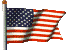Conflict Probability Estimation Generalized to Non-Level Flight

Author: Russell A. Paielli and Heinz Erzberger

Abstract: A method known as conflict probability estimation (CPE) is presented to estimate the probability of conflict (loss of separation) for pairs of aircraft with uncertain predicted trajectories. It is a generalization of a previous method that applied to level flight only. The generalized method is equivalent to the previous exact analytical solution for the special case of level flight, but it also gives an approximate analytical solution for non-level flight. The trajectory prediction errors are modeled as Gaussian, and the two error covariances for an aircraft pair are combined into a single, equivalent covariance of the relative position. A coordinate transformation is then used to derive the analytical solution. A Monte Carlo simulation demonstrates that the CPE algorithm is accurate, assuming the validity of the simplifying assumptions and the accuracy of the underlying prediction error model. Numerical examples are presented.

Reference: Air Traffic Control Quarterly, Vol. 7(3)(1999), pp 195-222

RussP.us